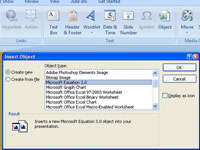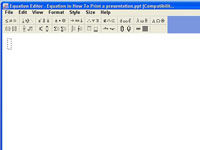Write Equations in PowerPoint 2007 - dummies

Use the Equation Editor to place mathematical equations and expressions on PowerPoint slides. The equations you draw become embedded PowerPoint objects so you can edit them without leaving PowerPoint, even though the Equation Editor is actually a separate program. Follow these steps to start PowerPoint Equation Editor and begin constructing an equation or expression on a PowerPoint slide:## 1Click the Insert tab.

It’s usually the second or third tab from the left in the Ribbon.## 2Click the Insert Object button.

The Insert Object dialog box opens.## 3Select Microsoft Equation 3.0 in the Object Type list and click OK.

The Equation Editor opens. You see the Equation toolbar, the menu bar, and a frame in which to write an expression. (If you don’t see the Equation toolbar, choose View→Toolbar.)